• 步骤1：定义问题 在组织或业务数据分析中，必须从正确的问题开始。问题应该是可衡量的，清晰明了的。定义问题，来帮助你获取解决特定问题或机会的潜在解决方案。 例如，从一个明确定义的问题开始：政
由于要整理的数据太多，因此我们需要从数据中获取更多准确的信息来帮助我们做出决策，那么：
你需要知道它是不是正确的数据；你需要根据这些数据得出准确的结论；你需要通过这些结论总结出正确的决策…
简而言之，你需要更好的进行数据分析来提高数据分析能力并简化决策。那么以下这5个步骤将会帮助你完成数据分析、制定决策方案：
一、步骤1：定义问题
在组织或业务数据分析中，必须从正确的问题开始。问题应该是可衡量的，清晰明了的。定义问题，来帮助你获取解决特定问题或机会的潜在解决方案。
例如，从一个明确定义的问题开始：政府承包商的成本不断上升，无法提交具有竞争力的合同建议。解决此业务问题的众多问题之一可能包括：公司可以在不影响质量的前提下减少人员吗？
二、步骤2：设定明确的测量优先级
此步骤分为两个子步骤：
1. 确定要测量的内容
以政府承包商为例，考虑一下回答关键问题需要什么样的数据。在这种情况下，需要知道当前员工的数量和成本以及他们花在必要业务职能上的时间百分比。
在回答这个问题时，可能需要回答许多子问题。
例如：人员当前是否被未充分利用？如果是，则哪些流程改进会有所帮助？
最后，在决定衡量标准时，请确保包括任何利益相关者可能提出的任何合理的反对意见，如：如果裁员，公司将如何应对需求激增？
2. 决定如何测量
考虑如何衡量数据也同样重要，尤其是在数据收集阶段之前。需要执行此步骤的关键问题包括：
时间是几点？（例如，年度费用与季度费用）计量单位是什么？（例如，美元兑欧元）应该包括哪些因素？（例如，仅是年薪vs年薪加上员工福利成本）
三、步骤3：收集资料
明确定义问题并确定测量优先级后，现在该收集数据了。在收集和整理数据时，请记住以下几点：
在收集新数据之前，请确定可以从现有数据库或现有资源中收集哪些信息，首先收集这些数据；提前确定文件存储和命名系统，以帮助所有任务小组成员进行协作。此过程可以节省时间并防止团队成员两次收集相同的信息；如果需要通过观察或访谈收集数据，请提前开发一个访谈模板以确保一致性并节省时间；将收集的数据整理成带有收集日期的日志，并随时添加任何源注释（包括执行的所有数据标准化），这种做法可以验证结论。
四、步骤4：分析资料
在收集了正确的数据以回答第1步中的问题之后，就该进行更深入的数据分析了。
首先以多种不同的方式来处理数据，例如将数据绘制出来并找到相关性，或者在Excel中创建数据透视表。通过数据透视表，可以按不同的变量对数据进行排序和过滤，并可以计算数据的平均值，最大值，最小值和标准偏差。
在此步骤中，数据分析工具和软件非常有用，Visio，Minitab和Stata都是用于高级统计数据分析的。
同样在大多数情况下，就决策工具而言，没有什么可以与Microsoft Excel相比。但是相对来说，Excel又过于简陋及简单，而编程工具又太过繁琐，那不如就使用数据可视化工具。
数据可视化工具，商业智能领域的一种，这就是为了数据分析而生的，而且它诞生的起点就非常高，目标是把从业务数据到经营决策的时间缩短，如何利用数据来影响决策。
五、步骤5：解读结果
在分析了数据并可能进行进一步的研究之后，现在是时候解释结果了。无论收集多少数据，机会总是会干扰结果。
在解释数据结果时，请问一问自己下面这些问题：
数据是否回答了原始问题？回答的怎么样？数据是否有助于打破异议？效果怎么样？结论是否有限制，是否有其他角度进行解读？
通过遵循数据分析过程中的这五个步骤，可以为企业或政府机构做出更好的决策，因为你的选择得到了经过可靠收集和分析的数据支持。
通过实践，数据分析将变得更快、更准确，这意味着将做出更好，更明智的决策，从而最有效地运营组织。
展开全文大数据 数据库 数据分析 数据挖掘
• 第一步建立模型;第二步使用模型;第四章 分类和预测;4.2 数据分类方法;分类过程的数据预处理;分类过程的数据预处理;分类过程的数据预处理;分类过程的数据预处理;分类过程的数据预处理;分类的评价标准;分类的评价标准;...
作者 | Revolver

一、具体代码和步骤
可视化我们的决策树模型的第一步是把模型训练出来。下面这个例子是在鸢尾花数据上，采用随机森林的方法训练出来的包含多棵决策树的模型，我们对其中一棵决策树进行可视化。
from sklearn.datasetsimport load_iris

# 导入随机森林模型 （此处也可换成决策树模型）
from sklearn.ensembleimport RandomForestClassifier
model =RandomForestClassifier(n_estimators=10)

# 训练
model.fit(iris.data,iris.target)

# 提取单棵决策树
tree =model.estimators_


训练好我们的模型之后，我们就可以用sklearn自带的export_graphviz函数将决策树模型转换成Graphviz格式。
from sklearn.treeimport export_graphviz

# 输出一个.dot格式的文件
export_graphviz(tree,out_file='tree.dot',
feature_names =iris.feature_names,
class_names =iris.target_names,
rounded = True, proportion =False,
precision = 2, filled = True)


参数含义:
precision 设置输出的纯度指标的数值精度
filled 指定是否为节点上色
max_depth 指定展示出来的树的深度，可以用来控制图像大小

有两种方式使用Graphviz这个工具，一种是执行pip install graphviz，然后可以在pyhton调用，另一种是使用命令行，这里我们采用第二种，但是是在python里调用的外部命令行。
# 临时将Graphviz添加到环境变量中
import os
os.environ["PATH"]+= os.pathsep + 'C:/Program Files (x86)/Graphviz2.38/bin/

# 将tree.dot文件转化为tree.png
from subprocess importcall
call(['dot', '-Tpng','tree.dot', '-o', 'tree.png', '-Gdpi=600'])

# 在jupyter notebook中查看决策树图像
from IPython.displayimport Image
Image(filename ='tree.png')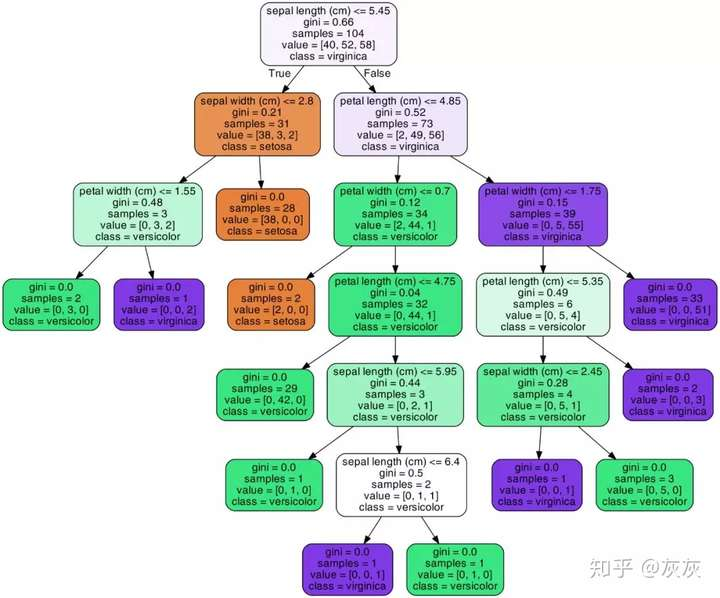上图就是我们将决策树可视化之后的结果，根据每个节点中的文字内容，我们就可以知道，这个节点包含的数据纯度大小（基尼指数或熵值），选用了哪个属性以及属性值对数据进行再划分，样本量多少，还可以根据节点颜色的深浅来推断类别，不同颜色代表不同类别，颜色深度越浅说明各个类别的混杂程度高，颜色越深说明纯度越高。上图中绿、紫、土黄三个颜色就表示了鸢尾花的三种类别。

通过这样的图，我们可以直观的展示随机森林中的每棵树，甚至推断每棵树形成背后的原因。

二、一个例子
这里我再举一个应用决策树可视化的例子。比如你想知道基于最小熵选择划分属性生成决策树这种方法不能产生一棵最小规模的决策树，即基于最大信息增益的原则去选择最优划分属性。这里我就用可视化决策树的方法来向大家说明这种方法是否可行。现在有下面这样一份数据：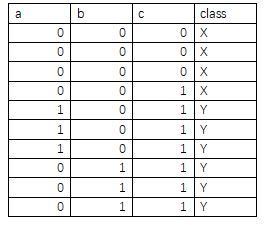其中a，b，c为三个属性，class为类别，分X，Y两种。在第一次对数据集进行划分时，根据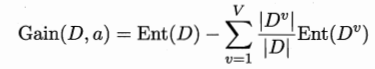计算属性的信息增益，得
Gain(D,c)=0.557，Gain(D,a)= Gain(D,b)= 0.2816
这里若基于熵最小方法首先应选择c作为划分属性，最后可得决策树可视化如下图。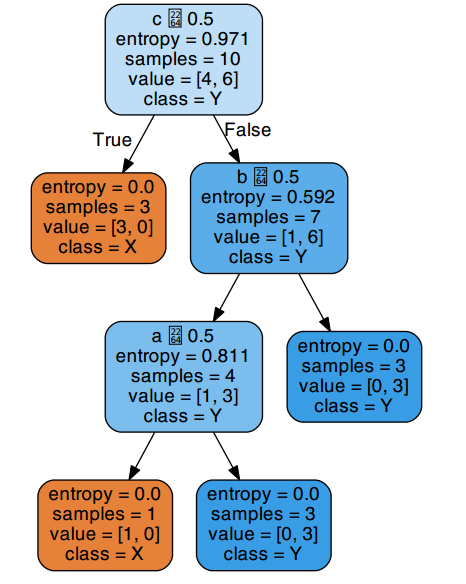在这棵树中，a,b,c三个属性全部被用来进行划分，产生了三个分支节点。但如果我们采用属性a作为第一个划分属性，会得到一棵这样的决策树：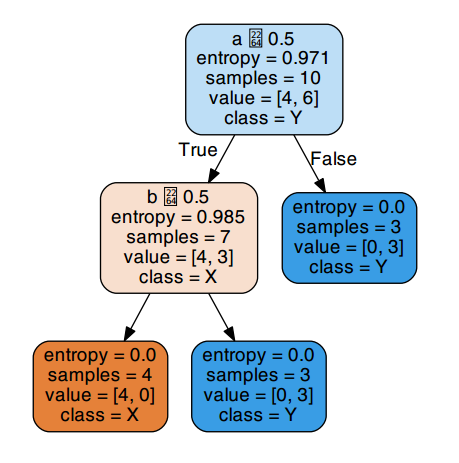由图可知，其实仅仅用到a,b两个属性即可将数据集完全划分为一颗叶子节点熵为0的完整决策树，这棵树只有两个分支节点，三个叶子结点，其规模显然小于上面的决策树。这个例子说明了基于最小熵的方法生成的决策树规模不一定就是最小的。它只能在局部范围内取得纯度最高的类别划分，是局部最优，从整个生成整棵决策树所有节点的过程来看，它并不能保证生成的节点数是最少的。
怎么样，上面这些例子中，决策树可视化是不是让一切看起来简单明了！

三、总结
现阶段机器学习模型仍然存在着黑盒问题，一张图片并不能彻底解决此问题。尽管如此，观察决策树图的过程还是向我们展示了这个模型并非一个不可解释的方法，而是一系列被提出的逻辑问题和答案，正如我们平时进行预测时所采用的步骤那样。所以，你可以直接复制上面的代码，尽情把这个工具用到你自己的数据集上，解决你的问题吧。
展开全文可视化
• 结点有两种类型：内部结点和叶结点，其中内部结点表示一个特征或属性，叶结点表示一个类。 分类树 分类树是种描述对实例进行分类的树形结构。 用决策树分类，从根结点开始，对实例的某特征进行测试，...
分类目录：《深入理解机器学习》总目录 相关文章： 基于决策树的模型（一）分类树和回归树 基于树的模型（二）：集成学习之Bagging和Random Forest 基于树的模型（三）：集成学习之GBDT和XGBoost 基于树的模型（四）：随机森林的延伸——深度森林（gcForest） 基于树的模型（五）：从零开始用Python实现ID3决策树 基于树的模型（六）：Python实现CART决策树并利用Tkinter构建GUI对决策树进行调优 基于树的模型（七）：RF/XGBoost等算法实践与决策树Scala实践等（材料准备中）

决策树（Decision Tree）是一种基本的分类与回归方法，当决策树用于分类时称为分类树，用于回归时称为回归树。本文主要讨论决策树中的分类树与回归树的一些基本理论，后续文章会继续讨论决策树的Boosting和Bagging相关方法。
决策树由结点和有向边组成。结点有两种类型：内部结点和叶结点，其中内部结点表示一个特征或属性，叶结点表示一个类。一般的，一棵决策树包含一个根结点、若干个内部结点和若干个叶结点。叶结点对应于决策结果，其他每个结点则对应于一个属性测试。每个结点包含的样本集合根据属性测试的结果被划分到子结点中，根结点包含样本全集，从根结点到每个叶结点的路径对应了一个判定测试序列。在下图中，圆和方框分别表示内部结点和叶结点。决策树学习的目的是为了产生一棵泛化能力强，即处理未见示例能力强的决策树。分类树
分类树是一种描述对实例进行分类的树形结构。在使用分类树进行分类时，从根结点开始，对实例的某一特征进行测试，根据测试结果，将实例分配到其子结点。这时，每一个子结点对应着该特征的一个取值。如此递归地对实例进行测试并分配，直至达到叶结点。最后将实例分到叶结点的类中。
假设给定训练数据集：

D

=

{

(

x

1

,

y

1

)

,

(

x

2

,

y

2

)

,

.

.

.

,

(

x

N

,

y

N

)

}

D=\{(x_1, y_1), (x_2, y_2), ..., (x_N, y_N)\}

其中，

x

i

=

(

x

i

(

1

)

,

x

i

(

2

)

,

.

.

.

,

x

i

(

n

)

)

T

,

x_i=(x_i^{(1)}, x_i^{(2)}, ..., x_i^{(n)})^T,

为输入实例，即特征向量，

n

n

为特征个数，

i

=

1

，

2

…

，

N

i=1，2…，N

，

N

N

为样本容量，

y

i

∈

{

1

,

2

,

.

.

.

,

K

}

y_i \in \{ 1, 2, ..., K\}

为类标。分类树学习的目标是根据给定的训练数据集构建一个决策树模型，使它能够对实例进行正确的分类。
决策树学习本质上是从训练数据集中归纳出一组分类规则。与训练数据集不相矛盾的决策树（即能对训练数据进行正确分类的决策树）可能有多个，也可能一个也没有。我们需要的是一个与训练数据矛盾较小的决策树，同时具有很好的泛化能力。从另一个角度看，决策树学习是由训练数据集估计条件概率模型。基于特征空间划分的类的条件概率模型有无穷多个，我们选择的条件概率模型应该不仅对训练数据有很好的拟合，而且对未知数据有很好的预测。
决策树学习用损失函数表示这一目标，其损失函数通常是正则化的极大似然函数，决策树学习的策略是以损失函数为目标函数的最小化。当损失函数确定以后，学习问题就变为在损失函数意义下选择最优决策树的问题。因为从所有可能的决策树中选取最优决策树是NP完全问题，所以现实中决策树学习算法通常采用启发式方法，近似求解这一最优化问题。这样得到的决策树是次最优的。

决策树分类算法 输入：

训练集：

D

=

(

x

1

,

y

1

)

,

(

x

2

,

y

2

)

,

⋯

,

(

x

N

,

y

N

)

D = {(x_1, y_1), (x_2, y_2), \cdots, (x_N, y_N)}

属性集：

A

=

a

1

,

a

2

,

⋯

,

a

n

A = {a_1, a_2, \cdots, a_n}

过程：

函数

T

r

e

e

G

e

n

e

r

a

t

e

(

D

,

A

)

TreeGenerate(D, A)

输出：

以node为根节点的决策树 算法： （ 1 ） 生成结点根node （ 2 ） if

D

D

中样本全属于同一类别

C

k

C_k

then （ 3 ）

将node标记为

C

k

C_k

类叶结点 （ 4 ）

return （ 5 ） end if （ 6 ） if

A

=

∅

A = \varnothing

OR

D

D

中样本在

A

A

上取值相同 then （ 7 ）

将node标记为叶结点，其类别标记为

D

D

中样本数最多的类 （ 8 ）

return （ 9 ）end if （10）从

A

A

中选择最优划分属性

a

∗

a_*

（11）for

a

∗

a_*

的每一个值

a

∗

v

a_*^v

do （12）

为node生成一个分支：令

D

v

D_v

表示

D

D

中在

a

∗

a_*

上取值为

a

∗

v

a_*^v

的样本子集 （13）

if

D

v

D_v

为空 then （14）

将分支结点标记为叶结点，其类别标记为

D

D

中样本最多的类 （15）

return （16）

else （17）

以

T

r

e

e

G

e

n

e

r

a

t

e

(

D

v

,

A

−

{

a

∗

}

)

TreeGenerate(D_v, A - \{a_*\})

为分支结点 （18）

end if （19）end for

决策树学习的算法通常是一个递归地选择最优特征，并根据该特征对训练数据进行分割，使得对各个子数据集有一个最好的分类的过程。这一过程对应着对特征空间的划分，也对应着决策树的构建。开始，构建根结点，将所有训练数据都放在根结点。选择一个最优特征，按照这一特征将训练数据集分割成子集，使得各个子集有一个在当前条件下最好的分类。如果这些子集已经能够被基本正确分类，那么构建叶结点，并将这些子集分到所对应的叶结点中去，如果还有子集不能被基本正确分类，那么就对这些子集选择新的最优特征，继续对其进行分割，构建相应的结点。如此递归地进行下去，直至所有训练数据子集被基本正确分类，或者没有合适的特征为止。最后每个子集都被分到叶结点上，即都有了明确的类。这就生成了一棵决策树。
从上述过程中就可以看出，决策树的生成是一个递归过程。在决策树基本算法中，有三种情形会导致递归返回
当前结点包含的样本全属于同一类别，无需划分当前属性集为空，或是所有样本在所有属性上取值相同，无法划分当前结点包含的样本集合为空，不能划分
在第二种情形下，我们把当前结点标记为叶结点，并将其类别设定为该结点所含样本最多的类别。在第三种情形下，同样把当前结点标记为叶结点，但将其类别设定为其父结点所含样本最多的类别。这两种情形的处理实质不同：第二种情况是在利用当前结点的后验分布，而第三种情况则是把父结点的样本分布作为当前结点的先验分布。
以上方法生成的决策树可能对训练数据有很好的分类能力，但对未知的测试数据却未必有很好的分类能力，即可能发生过拟合现象。我们需要对已生成的树自下而上进行剪枝，将树变得更简单，从而使它具有更好的泛化能力。具体地，就是去掉过于细分的叶结点，使其回退到父结点，甚至更高的结点，然后将父结点或更高的结点改为新的叶结点。如果特征数量很多，也可以在决策树学习开始的时候，对特征进行选择，只留下对训练数据有足够分类能力的特征。
可以看出，决策树学习算法包含特征选择、决策树的生成与决策树的剪枝过程。由于决策树表示一个条件概率分布，所以深浅不同的决策树对应着不同复杂度的概率模型。决策树的生成对应于模型的局部选择，决策树的剪枝对应于模型的全局选择。决策树的生成只考虑局部最优，相对地，决策树的剪枝则考虑全局最优。
决策树模型呈树形结构，在分类问题中，表示基于特征对实例进行分类的过程。它可以认为是if-then规则的集合，也可以认为是定义在特征空间与类空间上的条件概率分布。分类树具有良好的可读性与分类速度快的优点。分类树在学习时，利用训练数据，根据损失函数最小化的原则建立分类树模型，在预测时，对新的数据，利用分类树模型进行分类。决策树学习通常包括3个步骤：特征选择、决策树的生成和决策树的修剪。
决策树与if-then规则
可以将决策树看成一个if-then规则的集合：由决策树的根结点到叶结点的每一条路径构建一条规则，路径上内部结点的特征对应着规则的条件，而叶结点的类对应着规则的结论。决策树的路径或其对应的if-then规则集合具有一个重要的性质——互斥并且完备。这就是说，每一个实例都被一条路径或一条规则所覆盖，而且只被一条路径或一条规则所覆盖。这里所谓覆盖是指实例的特征与路径上的特征一致或实例满足规则的条件。
决策树与条件概率分布
决策树还表示给定特征条件下类的条件概率分布。这一条件概率分布定义在特征空间的一个划分上。将特征空间划分为互不相交的单元或区域，并在每个单元定义一个类的概率分布就构成了一个条件概率分布。决策树的一条路径对应于划分中的一个单元。决策树所表示的条件概率分布由各个单元给定条件下类的条件概率分布组成。假设

X

X

为表示特征的随机变量，

Y

Y

为表示类的随机变量，那么这个条件概率分布可以表示为

P

（

Y

∣

X

）

P（Y|X）

。

X

X

取值于给定划分下单元的集合，

Y

Y

取值于类的集合。各叶结点（单元）上的条件概率往往偏向某一个类，即属于某一类的概率较大。决策树分类时将该结点的实例强行分到条件概率大的那一类去。
决策树的优缺点
计算复杂度不高对中间缺失值不敏感解释性强，在解释性方面甚至比线性回归更强与传统的回归和分类方法相比，决策树更接近人的决策模式可以用图形表示，非专业人士也可以轻松理解可以直接处理定性的预测变量而不需创建哑变量决策树的预测准确性一般比回归和分类方法弱，但可以通过用集成学习方法组合大量决策树，显著提升树的预测效果
特征选择
特征选择在于选取对训练数据具有分类能力的特征。这样可以提高决策树学习的效率。如果利用一个特征进行分类的结果与随机分类的结果没有很大差别，则称这个特征是没有分类能力的。经验上扔掉这样的特征对决策树学习的精度影响不大。比如，我们希望构建一棵决策树来根据不同人的各种属性来预测每个人性别，那么对于属性“头发的长度”可能就要比属性“头发的颜色”所能包含的信息更多。因为一般来说，男生的头发要比女生的头发短，所以我们希望“头发的长度”这个属性处于决策树的上部。随着划分过程不断进行，我们希望决策树的分支结点所包含的样本尽可能属于同一类别，即结点的“纯度”（purity）越来越高。
信息增益
为了便于说明信息增益，先给出熵与条件熵的定义。在信息论与概率统计中，熵（entropy）是表示随机变量不确定性的度量。设

X

X

是一个取有限个值的离散随机变量，其概率分布为:

P

(

X

=

x

i

)

=

p

i

,

i

=

1

,

2

,

⋯

,

n

P(X = x_i) = p_i, i = 1, 2, \cdots, n

则随机变量

X

X

的熵定义为：

H

(

X

)

=

−

∑

i

=

1

n

p

i

log

⁡

p

i

H(X) = -\sum_{i = 1}^n p_i \log p_i

在上式中，若

p

i

=

0

p_i = 0

，则定义

p

i

log

⁡

p

i

=

0

p_i \log p_i = 0

。通常，上式中的对数以

2

2

为底或以

e

e

为底（自然对数），这时熵的单位分别称作比特（bit）或纳特（nat）.由定义可知，熵只依赖于

X

X

的分布，而与

X

X

的取值无关，所以也可将

X

X

的熵记作

H

(

p

)

H(p)

，即:

H

(

p

)

=

−

∑

i

=

1

n

p

i

log

⁡

p

i

H(p) = -\sum_{i = 1}^n p_i \log p_i

由此可见，熵越大，随机变量的不确定性就越大。从熵的定义可验证

0

≤

H

(

p

)

≤

log

⁡

n

0 \leq H(p) \leq \log n

当随机变量只取两个值，例如1，0时，即

X

X

的分布为:

P

(

X

=

1

)

=

p

,

P

(

X

=

0

)

=

1

−

p

,

0

≤

p

≤

1

P(X = 1) = p,\quad P(X = 0) = 1-p, \quad 0≤p≤1

其熵为：

H

(

p

)

=

−

p

log

⁡

2

p

−

(

1

−

p

)

log

⁡

2

(

1

−

p

)

H(p) = -p \log_2 p - (1 - p)\log_2 (1 - p)

这时，熵

H

(

p

)

H(p)

随概率

p

p

变化的曲线如下图所示（单位为比特）：当

p

=

0

p = 0

或

p

=

1

p = 1

时

H

(

p

)

=

0

H(p) = 0

，随机变量完全没有不确定，当

p

=

0.5

p = 0.5

时，

H

(

p

)

=

1

H(p) = 1

，熵取值最大，随机变量不确定性最大。
设有随机变量

(

X

,

Y

)

(X, Y)

，其联合概率分布为：

P

(

X

=

x

i

,

Y

=

y

i

)

=

p

i

j

{

i

=

1

,

2

,

⋯

,

n

j

=

1

,

2

,

⋯

,

m

P(X = x_i, Y = y_i) = p_{ij} \quad \begin{cases} i = 1, 2, \cdots, n \\ j = 1, 2, \cdots, m \end{cases}

条件熵

H

(

Y

∣

X

)

H(Y|X)

表示在已知随机变量

X

X

的条件下随机变量

Y

Y

的不确定性。随机变量

X

X

给定的条件下随机变量

Y

Y

的条件熵（conditional entropy）

H

(

Y

∣

X

)

H(Y|X)

，定义为

X

X

给定条件下

Y

Y

的条件概率分布的熵对

X

X

的数学期望：

H

(

Y

∣

X

)

=

∑

i

=

1

n

p

i

H

(

Y

∣

X

=

x

i

)

H(Y|X) = \sum_{i = 1}^n p_iH(Y|X = x_i)

其中，

p

i

=

P

(

X

=

x

i

)

,

i

=

1

,

2

,

⋯

,

n

p_i = P(X = x_i), i = 1, 2, \cdots, n

。
当熵和条件熵中的概率由数据估计（如极大似然估计）得到时，所对应的熵与条件熵分别称为经验熵（empirical entropy）和经验条件熵（empirical conditional entropy）。此时，如果有0概率，令

0

log

⁡

0

=

0

0\log0 = 0

。
信息增益（information gain）表示得知特征

X

X

的信息而使得类

Y

Y

的信息的不确定性减少的程度。特征

a

∗

a_*

对训练数据集

D

D

的信息增益

g

(

D

,

a

∗

)

g(D, a_*)

，定义为集合

D

D

的经验熵

H

(

D

)

H(D)

与特征

a

∗

a_*

给定条件下

D

D

的经验条件熵

H

(

D

∣

a

∗

)

H(D|a_*)

之差，即：

g

(

D

,

a

∗

)

=

H

(

D

)

−

H

(

D

∣

a

∗

)

g(D, a_*) = H(D) - H(D|a_*)

一般地，熵

H

(

Y

)

H(Y)

与条件熵

H

(

Y

∣

X

)

H(Y|X)

之差称为互信息（mutual information）。决策树学习中的信息增益等价于训练数据集中类与特征的互信息。
决策树学习应用信息增益准则选择特征。给定训练数据集

D

D

和特征

a

∗

a_*

，经验熵

H

(

D

)

H(D)

表示对数据集

D

D

进行分类的不确定性。而经验条件熵

H

(

D

∣

a

∗

)

H(D|a_*)

表示在特征

a

∗

a_*

给定的条件下对数据集

D

D

进行分类的不确定性。那么它们的差，即信息增益，就表示由于特征

a

∗

a_*

而使得对数据集

D

D

的分类的不确定性减少的程度。显然，对于数据集

D

D

而言，信息增益依赖于特征，不同的特征往往具有不同的信息增益，信息增益大的特征具有更强的分类能力。
根据信息增益准则的特征选择方法：对训练数据集（或子集）

D

D

，计算其每个特征的信息增益，并比较它们的大小，选择信息增益最大的特征。
设训练数据集为

D

D

，

∣

D

∣

|D|

表示其样本容量，即样本个数。设有

K

K

个类

C

k

C_k

，

k

=

1

,

2

,

⋯

,

K

k=1, 2, \cdots, K

，

∣

C

k

∣

|C_k|

为属于类

C

k

C_k

的样本个数，

∑

k

=

1

K

∣

C

k

∣

=

∣

D

∣

\sum_{k=1}^K |C_k| = |D|

。设特征

a

∗

a_*

有

V

V

个不同的取值

{

a

∗

1

,

a

∗

2

,

⋯

,

a

∗

V

}

\{ a_*^1, a_*^2, \cdots, a_*^V\}

，根据特征

a

∗

a_*

的取值将

D

D

划分为

V

V

个子集

D

1

,

D

2

,

⋯

,

D

V

D_1, D_2, \cdots, D_V

，

∣

D

t

∣

|D_t|

为

D

t

D_t

的样本个数，

∑

i

=

1

n

∣

D

t

∣

=

∣

D

∣

\sum_{i=1}^n|D_t|=|D|

。记子集

D

i

D_i

中属于类

C

k

C_k

的样本的集合为

D

i

k

D_{ik}

。即

D

i

k

=

D

i

∩

C

k

D_{ik} = D_i \cap C_k

，

∣

D

i

k

∣

|D_{ik}|

为

D

i

k

D_{ik}

的样本个数。于是计算信息增益的方法如下：

信息增益 输入：训练数据集

D

D

和特征

a

∗

a_*

输出：特征

a

∗

a_*

对训练数据集

D

D

的信息增益

g

(

D

,

a

∗

)

g(D, a_*)

1.计算数据集

D

D

的经验熵

H

(

D

)

H(D)

：

H

(

D

)

=

−

∑

k

=

1

K

C

k

D

log

⁡

2

C

k

D

H(D) = -\sum_{k=1}^K \frac{C_k}{D}\log_2\frac{C_k}{D}

2.计算特征

A

A

对数据集

D

D

的经验条件熵

H

(

D

∣

A

)

H(D|A)

：

H

(

D

∣

A

)

=

∑

i

=

1

n

∣

D

i

∣

D

H

(

D

i

)

=

−

∑

i

=

1

n

∣

D

i

∣

D

∑

k

=

1

K

D

i

k

D

i

log

⁡

2

D

i

k

D

i

H(D|A) = \sum_{i=1}^n\frac{|D_i|}{D}H(D_i)=-\sum_{i=1}^n\frac{|D_i|}{D}\sum_{k=1}^K\frac{D_{ik}}{D_i}\log_2\frac{D_{ik}}{D_i}

3.计算信息增益：

g

(

D

,

a

∗

)

=

H

(

D

)

−

H

(

D

∣

a

∗

)

g(D, a_*) = H(D) - H(D|a_*)

一般而言，信息增益越大，则意味着使用特征

a

∗

a_*

来进行划分所获得的“纯度提升”越大。因此，我们可用信息增益来进行决策树的划分属性选择，即在上述决策树分类算法第10行使用

a

∗

=

arg

max

⁡

a

∈

A

g

(

D

,

a

)

a_* = \text{arg}\ \max_{a \in A}g(D, a)

选择最优划分属性。著名的ID3决策树学习算法就是以信息增益为准则来选择划分属性。
ID3算法的核心是在决策树各个结点上应用信息增益准则选择特征，递归地构建决策树。具体方法是：从根结点开始，对结点计算所有可能的特征的信息增益，选择信息增益最大的特征作为结点的特征，由该特征的不同取值建立子结点。之后，再对子结点递归地调用以上方法，构建决策树，直到所有特征的信息增益均很小或没有特征可以选择为止，最终得到一个决策树。ID3相当于用极大似然法进行概率模型的选择。

ID3算法 输入：训练数据集

D

D

，特征集

A

A

，阈值

ϵ

\epsilon

输出：决策树

T

T

1.若

D

D

中所有实例属于同一类

C

k

C_k

，则

T

T

为单结点树，并将类

C

k

C_k

作为该结点的类标记，返回决策树

T

T

2.若

A

=

∅

A = \varnothing

，则

T

T

为单结点树，并将

D

D

中实例数最大的类

C

k

C_k

，作为该结点的类标记，返回决策树

T

T

3.否则，计算

A

A

中各特征对

D

D

的信息增益，选择信息增益最大的特征

a

∗

a_*

4.如果

a

∗

a_*

的信息增益小于阈值

ϵ

\epsilon

，则置

T

T

为单结点树，并将

D

D

中实例数最大的类

C

k

C_k

作为该结点的类标记，返回决策树

T

T

5.否则，对

a

∗

a_*

的每一可能值

a

∗

v

a_*^v

，依

a

∗

=

a

∗

v

a_* = a_*^v

将

D

D

分割为若干非空子集

D

v

D_v

，将

D

v

D_v

中实例数最大的类作为标记，构建子结点，由结点及其子结点构成决策树

T

T

，返回决策树

T

T

6.对第

v

v

个子结点，以

D

v

D_v

为训练集，以

A

−

{

a

∗

}

A - \{a_*\}

为特征集，递归地调用第(1)步~第(5)步，得到子树

T

v

T_v

，并返回子树

T

v

T_v

信息增益率
信息增益值的大小是相对于训练数据集而言的，并没有绝对意义。在训练数据集的经验熵大的时候，信息增益值会偏大。反之，信息增益值会偏小。使用信息增益率（information gain ratio）可以对这一问题进行校正。这是特征选择的另一准则。特征

a

∗

a_*

对训练数据集

D

D

的信息增益率

g

g

(

D

,

a

∗

)

g_g(D, a_*)

定义为其信息增益

g

(

D

,

a

∗

)

g(D, a_*)

与训练数据集

D

D

的经验熵

H

(

D

)

H(D)

之比：

g

g

(

D

,

a

∗

)

=

g

(

D

,

a

∗

)

H

(

D

)

g_g(D, a_*) = \frac{g(D, a_*)}{H(D)}

如前文所说，信息增益准则对可取值数目较多的属性有所偏好，为减少这种偏好可能带来的不利影响，著名的C4.5决策树算法不直接使用信息增益来选择划分属性，而是使用信息增益率来选择最优划分属性。

C4.5算法 输入：训练数据集

D

D

，特征集

A

A

，信息增益率阈值

ϵ

\epsilon

，信息增益阈值

α

\alpha

输出：决策树

T

T

1.若

D

D

中所有实例属于同一类

C

k

C_k

，则

T

T

为单结点树，并将类

C

k

C_k

作为该结点的类标记，返回决策树

T

T

2.若

A

=

∅

A = \varnothing

，则

T

T

为单结点树，并将

D

D

中实例数最大的类

C

k

C_k

，作为该结点的类标记，返回决策树

T

T

3.否则，计算

A

A

中各特征对

D

D

的信息增益和信息增益率，在信息增益大于

α

\alpha

的特征中选择信息增益率最大的特征

a

∗

a_*

4.如果

a

∗

a_*

的信息增益率小于阈值

ϵ

\epsilon

，则置

T

T

为单结点树，并将

D

D

中实例数最大的类

C

k

C_k

作为该结点的类标记，返回决策树

T

T

5.否则，对

a

∗

a_*

的每一可能值

a

∗

v

a_*^v

，依

a

∗

=

a

∗

v

a_* = a_*^v

将

D

D

分割为若干非空子集

D

v

D_v

，将

D

v

D_v

中实例数最大的类作为标记，构建子结点，由结点及其子结点构成决策树

T

T

，返回决策树

T

T

6.对第

v

v

个子结点，以

D

v

D_v

为训练集，以

A

−

{

a

∗

}

A - \{a_*\}

为特征集，递归地调用第(1)步~第(5)步，得到子树

T

v

T_v

，并返回子树

T

v

T_v

需注意的是，信息增益率准则对可取值数目较少的属性有所偏好，因此，C4.5算法并不是直接选择增益率最大的候选划分属性，而是使用了一个启发式的方法选择最优划分属性：先从候选划分属性中找出信息增益高于平均水平的属性，再从中选择增益率最高的.。
连续值处理
实际的任务中常会遇到连续属性，对于全部为连续属性的样本来说，我们一般使用回归决策树来处理。C4.5算法则采用了二分法对连续属性进行处理。由于连续属性的可取值数目不再有限，因此，不能直接根据连续属性的可取值来对结点进行划分。此时，连续属性离散化技术可派上用场。最简单的策略是采用二分法对连续属性进行处理。
给定样本集

D

D

和连续属性

a

a

，假定

a

a

在

D

D

上出现了

n

n

个不同的取值，将这些值从小到大进行排序，记为

{

a

1

,

a

2

,

a

3

,

⋯

,

a

n

}

\{a_1, a_2, a_3, \cdots, a_n\}

。基于划分点

t

t

可将

D

D

分为子集

D

t

+

D_t^+

和

D

t

−

D_t^-

，其中

D

t

+

D_t^+

包含那些在属性

a

a

上取值大于

t

t

的样本，而

D

t

−

D_t^-

则包含那些在属性

a

a

上取值不大于

t

t

的样本。显然，对相邻的属性取值

a

i

a^i

与

a

i

+

1

a^{i + 1}

来说，

t

t

在区间

[

a

i

,

a

i

+

1

)

[a^i, a^{i + 1})

中取任意值所产生的划分结果相同.因此，对连续属性

a

a

，我们可考察包含

n

−

1

n-1

个元素的候选划分点集合：

T

a

=

{

a

i

+

a

i

+

1

2

∣

1

≤

i

≤

n

−

1

}

T_a = \{\frac{a^i + a^{i + 1}}{2} \ | \ 1 \leq i \leq n - 1\}

即把区间

[

a

i

,

a

i

+

1

)

[a^i, a^{i + 1})

的中位点

a

i

+

a

i

+

1

2

\frac{a^i + a^{i + 1}}{2}

作为候选划分点。然后，我们就可像离散属性值一样来考察这些划分点，选取最优的划分点进行样本集合的划分：

G

a

i

n

(

D

,

a

)

=

max

⁡

t

∈

T

a

G

a

i

n

(

D

,

a

,

t

)

=

max

⁡

t

∈

T

a

E

n

t

(

D

)

−

∑

λ

∈

{

−

,

+

}

D

t

λ

D

E

n

t

(

D

t

λ

)

\begin{aligned} Gain(D, a) &= \max_{t \in T_a} Gain(D, a, t)\\ & = \max_{t \in T_a} Ent(D) - \sum_{\lambda \in \{-, +\}} \frac{D^\lambda _t}{D}Ent(D^\lambda _t) \end{aligned}

其中

G

a

i

n

(

D

,

a

,

t

)

Gain(D, a, t)

是样本集

D

D

基于划分点

t

t

二分后的信息增益。于是，我们就可选择使

G

a

i

n

(

D

,

a

,

t

)

Gain(D, a, t)

最大化的划分点。
缺失值处理
现实任务中常会遇到不完整样本，即样本的某些属性值缺失。且在属性数目较多的情况下，有时会有大量样本出现缺失值。如果简单地放弃不完整样本，仅使用无缺失值的样本来进行学习，显然是对数据信息极大的浪费。显然，有必要考虑利用有缺失属性值的训练样例来进行学习。
划分属性的选择
给定训练集

D

D

和属性

a

a

，令

D

~

\tilde{D}

表示

D

D

中在属性

a

a

上没有缺失值的样本子集。显然，我们仅可根据

D

~

\tilde{D}

来判断属性

a

a

的优劣。假定属性

a

a

有

V

V

个可取值

{

a

1

,

a

2

,

a

3

,

⋯

,

a

V

}

\{a^1, a^2, a^3, \cdots, a^V\}

，令

D

~

v

\tilde{D}^v

表示

D

~

\tilde{D}

中在属性

a

a

上取值为

a

v

a^v

的样本子集，

D

~

k

\tilde{D}_k

表示

D

~

\tilde{D}

中属于第

k

k

类

(

k

=

1

,

2

,

3

,

⋯

,

K

)

(k = 1, 2, 3, \cdots, K)

的样本子集，则显然有

D

~

=

⋃

k

=

1

K

D

~

k

\tilde{D} = \bigcup^K_{k = 1}\tilde{D}_k

与

D

~

=

⋃

v

=

1

V

D

~

v

\tilde{D} = \bigcup^V_{v = 1}\tilde{D}^v

。假定我们为每个样本

x

x

赋予一个权重

ω

x

\omega_x

，并定义：

ρ

=

∑

x

∈

D

~

ω

x

∑

x

∈

D

ω

x

p

~

k

=

∑

x

∈

D

~

k

ω

x

∑

x

∈

D

~

ω

x

r

~

v

=

∑

x

∈

D

~

v

ω

x

∑

x

∈

D

~

ω

x

\begin{aligned} \rho & = \frac{\sum_{x \in \tilde{D}}\omega_x}{\sum_{x \in D}\omega_x}\\ \tilde{p}_k & = \frac{\sum_{x \in \tilde{D}_k}\omega_x}{\sum_{x \in \tilde{D}}\omega_x}\\ \tilde{r}_v & = \frac{\sum_{x \in \tilde{D}^v}\omega_x}{\sum_{x \in \tilde{D}}\omega_x} \end{aligned}

直观地看，对于属性

a

a

，

ρ

\rho

表示无缺失值样本所占的比例，

p

~

k

\tilde{p}_k

表示无缺失值样本中第

k

k

类所占的比例，

r

~

v

\tilde{r}_v

则表示无缺失值样本中在属性

a

a

上取值

a

v

a^v

的样本所占的比例。基于上述定义，我们可将信息增益的计算式推广为：

G

a

i

n

(

D

,

a

)

=

ρ

×

G

a

i

n

(

D

~

,

a

)

=

ρ

×

(

E

n

t

(

D

~

)

−

∑

x

=

1

V

r

~

v

E

n

t

(

D

~

v

)

)

\begin{aligned} Gain(D, a) &= \rho \times Gain(\tilde{D}, a)\\ & = \rho \times (Ent(\tilde{D}) - \sum_{x = 1}^V \tilde{r}_v Ent(\tilde{D}^v)) \end{aligned}

对样本进行划分
根据上面的定义，若样本

x

x

在划分属性

a

a

上的取值已知，则将

x

x

划入与其取值对应的子结点，且样本权值在子结点中保持为

ω

x

\omega_x

。若样本

x

x

在划分属性

a

a

上的取值未知，则将

x

x

同时划入所有子结点，且样本权值在与属性值

a

v

a^v

对应的子结点中调整为

r

~

v

×

ω

x

\tilde{r}_v \times \omega_x

。直观地看，这就是让同一个样本以不同的概率划入到不同的子结点中去。
基尼指数
数据集

D

D

的纯度还可用基尼值来度量：

G

i

n

i

(

D

)

=

∑

k

=

1

K

p

k

(

1

−

p

k

)

=

1

−

∑

k

=

1

K

p

k

2

Gini(D) = \sum^K_{k=1}p_k(1 - p_k) = 1 - \sum^K_{k=1}p_k^2

其中，

K

K

为类别数，

p

k

p_k

为样本点属于第

k

k

类的概率。对于二类分类问题，若样本点属于第1个类的概率是

p

p

，则概率分布的基尼指数为：

G

i

n

i

(

D

)

=

2

p

(

1

−

p

)

Gini(D) = 2p(1 - p)

直观来说，

G

i

n

i

(

D

)

Gini(D)

反映了从数据集

D

D

中随机抽取两个样本，其类别标记不一致的概率。因此，

G

i

n

i

(

D

)

Gini(D)

越小，则数据集

D

D

的纯度越高。对于属性

a

a

的基尼指数定义为:

G

i

n

i

_

i

n

d

e

x

(

D

,

a

)

=

∑

v

=

1

V

D

v

D

G

i

n

i

(

D

v

)

Gini\_index(D, a) = \sum^V_{v = 1}\frac{D^v}{D}Gini(D^v)

在CART算法中，我们在候选属性集合

A

A

中，选择那个使得划分后基尼指数最小的属性作为最优划分属性，即：

a

∗

=

arg

min

⁡

a

∈

A

G

i

n

i

_

i

n

d

e

x

(

D

,

a

)

a_* = \text{arg}\min_{a \in A}Gini\_index(D, a)

在二类分类问题中，基尼指数

G

i

n

i

(

D

)

Gini(D)

、熵

H

(

p

)

H(p)

的一半，和分类误差率的关系：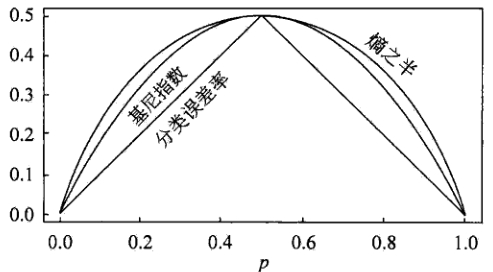其中，横坐标表示概率

p

p

，纵坐标表示损失。可以看出基尼指数和熵的一半的曲线很接近，都可以近似地代表分类误差率。
分类树的剪枝
剪枝（pruning）是决策树学习算法对付“过拟合”的主要手段。在决策树学习中，为了尽可能正确分类训练样本，结点划分过程将不断重复，有时会造成决策树分支过多，这时就可能因为对训练样本学习得“太好”了，以致于把训练集自身的一些特点当作所有数据都具有的一般性质而导致过拟合。因此，可通过主动去掉一些分支来降低过拟合的风险。决策树剪枝的基本策略有预剪枝和后剪枝。
预剪枝
预剪枝是指在决策树生成过程中，对每个结点在划分前先进行估计，若当前结点的划分不能带来决策树泛化性能提升，则停止划分并将当前结点标记为叶结点。停止决策树生长常用方法：
定义一个高度，当决策树达到该高度时就停止决策树的生长达到某个节点的实例具有相同的特征向量，即使这些实例不属于同一类，也可以停止决策树的生长。这个方法对于处理数据的数据冲突问题比较有效。定义一个阈值，当达到某个节点的实例个数小于阈值时就可以停止决策树的生长定义一个阈值，通过计算每次扩张对系统性能的增益，并比较增益值与该阈值大小来决定是否停止决策树的生长。
后剪枝
后剪枝则是先从训练集生成一棵完整的决策树，然后自底向上地对非叶结点进行考察，若将该结点对应的子树替换为叶结点能带来决策树泛化性能提升，则将该子树替换为叶结点。相比于预剪枝，后剪枝更常用，因为在预剪枝中精确地估计何时停止树增长很困难。
错误率降低剪枝（REP，Reduced-Error Pruning）
错误率降低剪枝方法是一种比较简单的后剪枝的方法。在该方法中，可用的数据被分成两个样例集合：首先是训练集，它被用来形成学习到的决策树，另一个是与训练集分离的验证集，它被用来评估这个决策树在后续数据上的精度，确切地说是用来评估修剪这个决策树的影响。学习器可能会被训练集中的随机错误和巧合规律所误导，但验证集合不大可能表现出同样的随机波动。所以验证集可以用来对过度拟合训练集中的虚假特征提供防护检验。
错误率降低剪枝方法考将树上的每个节点作为修剪的候选对象，再决定是对该节点进行剪枝：
删除以此结点为根的子树使其成为叶子结点当修剪后的树对于验证集合的性能不比修剪前的树的性能差时，则确认删除该结点，否则恢复该节点
因为训练集合的过拟合，使得验证集合数据能够对其进行修正，反复进行上面的操作，从底向上的处理结点，删除那些能够提高验证集合的精度的结点，直到进一步修剪会降低验证集合的精度为止。
错误率降低剪枝方法是最简单的后剪枝方法之一，不过由于使用独立的测试集，原始决策树相比，修改后的决策树可能偏向于过度修剪。这是因为一些不会再次在测试集中出现的很稀少的训练集实例所对应的分枝在剪枝过程中往往会被剪枝。尽管错误率降低剪枝方法有这个缺点，不过错误率降低剪枝方法仍然作为一种基准来评价其它剪枝算法的性能。它对于两阶段决策树学习方法的优点和缺点提供了一个很好的学习思路。由于验证集合没有参与决策树的构建，所以用错误率降低剪枝方法剪枝后的决策树对于测试样例的偏差要好很多，能够解决一定程度的过拟合问题。
悲观错误剪枝（PEP，Pesimistic-Error Pruning）
悲观错误剪枝方法是根据剪枝前后的错误率来判定子树的修剪。它不需要像错误率降低修剪方法那样，需要使用部分样本作为测试数据，而是完全使用训练数据来生成决策树，并进行剪枝，即决策树生成和剪枝都使用训练集。
该方法引入了统计学中连续修正的概念弥补错误率降低剪枝方法中的缺陷，在评价子树的训练错误公式中添加了一个常数，即假定每个叶子结点都自动对实例的某个部分进行错误的分类。
把一颗具有多个叶子节点的子树的分类用一个叶子节点来替代的话，在训练集上的误判率肯定是上升的，但是在新数据上不一定。于是我们把子树的误判计算加上一个经验性的惩罚因子来做是否剪枝的考量指标。对于一个叶子节点，它覆盖了

N

N

个样本，其中有

E

E

个错误，那么该叶子节点的错误率为

E

+

0.5

N

\frac{E + 0.5}{N}

。这个

0.5

0.5

就是惩罚因子，那么一颗子树，它有

L

L

个叶子节点，那么该子树的误判率估计为：

∑

E

i

+

0.5

∗

L

∑

N

i

\frac{\sum E_i +0.5 * L}{\sum N_i}

这样的话，我们可以看到一棵子树虽然具有多个子节点，但由于加上了惩罚因子，所以子树的误判率计算未必占到便宜。剪枝后内部节点变成了叶子节点，其误判个数

E

E

也需要加上一个惩罚因子，变成

E

+

0.5

E+0.5

，那么子树是否可以被剪枝就取决于剪枝后的错误

E

+

0.5

E+0.5

在的标准误差内。对于样本的误差率

e

e

，我们可以根据经验把它估计成各种各样的分布模型，比如二项式分布、正态分布等。如果

E

+

0.5

<

E

i

+

S

E

(

E

i

)

E+0.5 < E_i + SE(E_i)

则对

i

i

进行剪枝。
代价复杂度剪枝（CCP，Cost-Complexity Pruning)
代价复杂度剪枝算法为子树

T

t

T_t

定义了代价和复杂度，以及一个可由用户设置的衡量代价与复杂度之间关系的参数

α

α

。其中，代价指在剪枝过程中因子树

T

t

T_t

被叶节点替代而增加的错分样本，复杂度表示剪枝后子树

T

t

T_t

减少的叶结点数，

α

α

则表示剪枝后树的复杂度降低程度与代价间的关系，定义为：

α

=

R

(

t

)

−

R

(

T

t

)

∣

N

t

∣

−

1

\alpha = \frac{R(t) - R(T_t)}{|N_t| - 1}

其中，

∣

N

t

∣

|N_t|

是子树

T

t

T_t

中的叶节点数，

R

(

t

)

=

r

(

t

)

∗

p

(

t

)

R(t) = r(t) * p(t)

为结点

t

t

的错误代价，

r

(

t

)

r(t)

为结点

t

t

的错分样本率，

p

(

t

)

p(t)

为落入结点

t

t

的样本占所有样本的比例，

R

(

T

t

)

=

∑

R

(

i

)

R(T_t) = \sum R(i)

是子树

T

t

T_t

错误代价，

i

i

为子树

T

t

T_t

的叶节点。
对于完全决策树

T

T

的每个非叶结点计算

α

α

值，循环剪掉具有最小

α

α

值的子树，直到剩下根节点，得到一系列的剪枝树

{

T

0

,

T

‘

1

,

T

2

,

⋯

,

T

m

}

\{ T_0, T_1, T_2, \cdots, T_m \}

，其中

T

0

T_0

为原有的完全决策树，

T

m

T_m

为根结点，

T

i

+

1

T_{i +1}

为对

T

i

T_i

进行剪枝的结果从子树序列中，根据真实的误差估计选择最佳决策树
REPPEPCCP剪枝方式自底向上自顶向下自底向上计算复杂度

O

(

n

)

O(n)

O

(

n

)

O(n)

O

(

n

2

)

O(n^2)

误差估计测试集上误差估计使用连续纠正标准误差
回归树
建立回归树的过程大致可以分为两步：
将预测变量空间（

X

1

,

X

2

,

X

3

,

⋯

,

X

p

X_1, X_2, X_3, \cdots, X_p

）的可能取值构成的集合分割成

J

J

个互不重叠的区域

{

R

1

,

R

2

,

R

3

,

⋯

,

R

J

}

\{ R_1, R_2, R_3, \cdots, R_J\}

对落入区域

R

j

R_j

的每个观测值作同样的预测，预测值等于

R

j

R_j

上训练集的各个样本取值的算术平均数。
比如，在第一步中得到两个区域

R

1

R_1

和

R

2

R_2

，

R

1

R_1

中训练集的各个样本取值的算术平均数为10，

R

2

R_2

中训练集的各个样本取值的算术平均数为20。则对给定的观测值

X

=

x

X = x

，若

x

∈

R

1

x \in R_1

，给出的预测值为10，若

x

∈

R

2

x \in R_2

，则预测值为20。
所以，类似于上述决策树分类算法的第(10)步，关键在于如何构建区域划分

{

R

1

,

R

2

,

R

3

,

⋯

,

R

J

}

\{ R_1, R_2, R_3, \cdots, R_J\}

。事实上，区域的形状是可以为任意形状的，但出于模型简化和增强可解释性的考虑，这里将预测变量空间划分成高维矩形，我们称这些区域为称盒子。划分区域的目标是找到使模型的残差平方和

R

S

S

最小的矩形区域

{

R

1

,

R

2

,

R

3

,

⋯

,

R

J

}

\{ R_1, R_2, R_3, \cdots, R_J\}

。

R

S

S

的定义为：

R

S

S

=

∑

j

=

1

J

∑

i

∈

R

j

(

y

i

−

y

^

R

j

)

2

RSS = \sum^J_{j=1} \sum_{i \in R_j}(y_i - \hat{y}_{R_j})^2

其中，

y

^

R

j

\hat{y}_{R_j}

是第

j

j

个矩形区域中训练集中各个样本取值的算术平均数。但是，要想考虑将特征空间划分为

J

J

个矩形区域的所有可能性，在计算上是不可行的。因此一般采用一种自上而下的贪婪法：递归二又分裂。“自上而下”指的是它从树顶端开始依次分裂预测变量空间，每个分裂点都产生两个新的分支。“贪婪”意指在建立树的每一步中，最优分裂确定仅限于某一步进程，而不是针对全局去选择那些能够在未来进程中构建出更好的树的分裂点。
在执行递归二又分裂时，先选择预测变量

X

j

X_j

和分割点

s

s

，将预测变量空间分为两个区域

{

X

∣

X

j

<

s

}

\{ X|X_j <s \}

和

{

X

∣

X

j

≥

s

}

\{ X|X_j \geq s \}

，使

R

S

S

尽可能地减小。也就是说，考虑所有预测变量

X

1

,

X

2

,

X

3

,

⋯

,

X

p

X_1, X_2, X_3, \cdots, X_p

和与每个预测变量对应的

s

s

的取值，然后选择预测变量和分割点，使构造出的树具有最小的

R

S

S

。更详细地，对

j

j

和

s

s

，定义一对半平面：

R

1

(

j

,

s

)

=

{

X

∣

X

j

<

s

}

和

R

2

(

j

,

s

)

=

{

X

∣

X

j

≥

s

}

R_1(j, s) = \{ X|X_j <s \} \quad \text{和} \quad R_2(j, s) = \{ X|X_j \geq s \}

寻找

j

j

和

s

s

，使得下式最小：

∑

x

i

∈

R

1

(

j

,

s

)

(

y

i

−

y

^

R

1

)

2

+

∑

x

i

∈

R

2

(

j

,

s

)

(

y

i

−

y

^

R

2

)

2

\sum_{x_i \in R_1(j, s)}(y_i - \hat{y}_{R_1})^2 + \sum_{x_i \in R_2(j, s)}(y_i - \hat{y}_{R_2})^2

重复上述步骤，寻找继续分割数据集的最优预测变量和最优分割点，使随之产生的区域中的

R

S

S

达到最小。此时被分割的不再是整个预测变量空间，而是之前确定的两个区域之一。如此一来就能得到3个区域。接着进一步分割3个区域之一以最小化

R

S

S

。这一过程不断持续，直到符合某个停止准则，如我们在分类决策树中讨论到的前剪枝中的停止准则。
区域

{

R

1

,

R

2

,

R

3

,

⋯

,

R

J

}

\{ R_1, R_2, R_3, \cdots, R_J\}

产生后，就可以确定某一给定的测试数据所属的区域，并用这一区域训练集的各个样本取值的算术平均数作为与测试进行预测。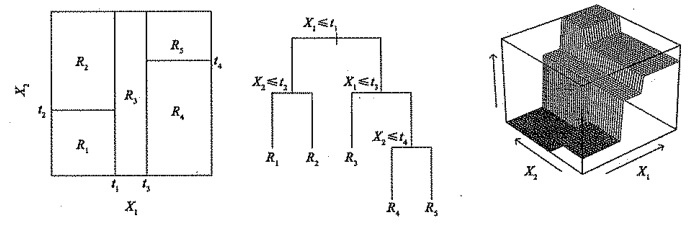回归树的剪枝
上述方法生成的回归树会在训练集中取得良好的预测效果，却很有可能造成数据的过拟合，导致在测试集上效果不佳。原因在于这种方法产生的树可能过于复杂。一棵分裂点更少、规模更小（区域

{

R

1

,

R

2

,

R

3

,

⋯

,

R

J

}

\{ R_1, R_2, R_3, \cdots, R_J\}

的个数更少）的树会有更小的方差和更好的可解释性（以增加微小偏差为代价）。针对上述问题，一种可能的解决办法是：仅当分裂使残差平方和

R

S

S

的减小量超过某阈值时，才分裂树结点。这种策略能生成较小的树，但可能产生过于短视的问题，一些起初看来不值得的分裂却可能之后产生非常好的分裂。也就是说在下一步中，

R

S

S

会大幅减小。
因此，更好的策略是生成一棵很大的树

T

0

T_0

α

\alpha

标记的一系列子树。每一个

α

\alpha

的取值对应一棵子树

T

∈

T

0

T \in T_0

，当

α

\alpha

一定时，其对应的子树使下式最小：

∑

m

=

1

∣

T

∣

∑

x

i

∈

R

m

(

y

i

−

y

^

R

m

)

2

+

α

∣

T

∣

\sum_{m=1}^{|T|} \sum_{x_i \in R_m}(y_i - \hat{y}_{R_m})^2 + \alpha |T|

这里的

∣

T

∣

|T|

表示树

T

T

的结点数，

R

m

R_m

是第

m

m

个终端结点对应的矩形（预测向量空间的一个子集），

y

^

R

m

\hat{y}_{R_m}

是与

R

m

R_m

对应的预测值，也就是

R

m

R_m

中训练集的平均值。调整系数

α

\alpha

在子树的复杂性和与训练数据的契合度之间控制权衡。当

α

=

0

\alpha = 0

时，子树

T

T

等于原树

T

0

T_0

，因为此时上式只衡量了训练误差。而当

α

\alpha

增大时，终端结点数多的树将为它的复杂付出代价，所以使上式取到最小值的子树会变得更小。
当

α

\alpha

从0开始逐渐增加时，树枝以一种嵌套的、可预测的模式被修剪，因此获得与

α

\alpha

对应的所有子树序列是很容易的。可以用交又验证或验证集确定

α

\alpha

，然后在整个数据集中找到与之对应的子树：

回归决策树算法 1.利用递归二叉分裂在训练集中生成一额大树，只有当终端结点包含的观测值个数低于某个最小值时才停止。 2.对大树进行代价复杂性剪枝，得到一系列最优子树，子树是

α

\alpha

的函数。 3.利用

K

K

折交叉验诞选择

α

\alpha

。具体做法是将训练集分为

K

K

折。对所有

k

=

1

,

2

,

3

,

⋯

,

K

k=1, 2, 3, \cdots, K

，对训练集上所有不属于第

k

k

折的数据重复第(1)步~第(2)步得到与

α

\alpha

对应的子树，并求出上述子树在第

k

k

折上的均方预测误差。 4.每个

α

\alpha

会有相应的

K

K

个均方预测误差，对这

K

K

个值求平均，选出使平均误差最小的

α

\alpha

。 5.找出选定的

α

\alpha

在第(2)步中对应的子树。

决策树的基础即是上文所述的分类决策树与回归决策树，其预测准确性一般比回归和分类方法弱，但可以通过用集成学习方法组合大量决策树，显著提升树的预测效果，这些方法我将会在接下来的文章中继续阐述，欢迎关注学习讨论！
展开全文机器学习 cart分类回归树 回归树
• ## 决策树与随机森林(从入门到精通)

万次阅读 多人点赞 2020-07-06 22:54:44
决策树（decision tree）是种...决策树学习通常包括三个步骤：特征选择，决策树的生成和决策树的修剪。而随机森林则是由多个决策树所构成的种分类器，更准确的说，随机森林是由多弱分类器组合形成的强分类器。
  决策树（decision tree）是一种基本的分类与回归方法，本文主要讨论用于分类的决策树。决策树学习通常包括三个步骤：特征选择，决策树的生成和决策树的修剪。而随机森林则是由多个决策树所构成的一种分类器，更准确的说，随机森林是由多个弱分类器组合形成的强分类器。

目录
一、信息论知识1.信息熵的概念2.条件熵3.相对熵4.互信息5.几个量之间的关系
二、决策树1.引入2.决策树的生成算法1.信息增益与ID32.信息增益率与C4.53.Gini系数与CART
3.决策树的评价4.决策树的过拟合1.剪枝

三、随机森林1.Bootstrap2.bagging策略3.out of bag estimate（包外估计）4.样本随机与特征随机

一、信息论知识
1.信息熵的概念
设离散型随机变量X的取值有

x

1

,

x

2

,

x

3

,

.

.

.

,

x

n

x_{1},x_{2},x_{3},...,x_{n}

，其发生概率分别为

p

1

,

p

2

,

p

3

,

.

.

.

,

p

n

p_{1},p_{2},p_{3},...,p_{n}

，那么就可以定义信息熵为：一般对数的底数是2，当然也可以换成e，当对数底数为2时，信息熵的单位为比特，信息熵也称香农熵。当对数不为2而是其他大于2的整数r时，我们称信息熵为r-进制熵，记为

H

r

(

X

)

H_{r}(X)

，它与信息熵转换公式为：信息熵用以描述信源的不确定度， 概率越大，可能性越大，但是信息量越小，不确定性越小，熵越小。
2.条件熵
设随机变量(X,Y)具有联合概率分布：条件熵

H

(

Y

∣

X

)

H(Y|X)

表示 在已知随机变量X的条件下随机变量Y的不确定性。    可以这样理解：    (X,Y)发生所包含的熵，减去X单独发生的熵，就是在X发生的前提下，Y发生新带来的熵。    所以条件熵有如下公式成立：推导如下：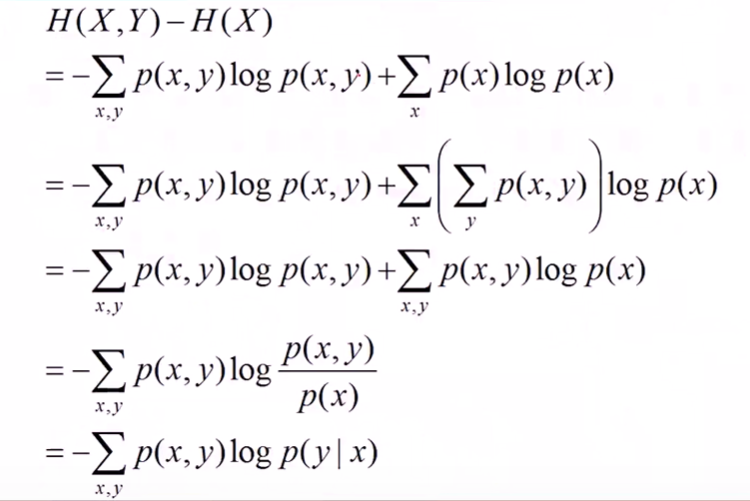3.相对熵
相对熵，又称互熵，交叉熵，鉴别信息，Kullback熵，Kullback-Leible散度等。定义如下： 设p(x)，q(x)是随机变量X中取值的两个概率分布，则p对q的相对熵为：在信息理论中，相对熵等价于两个分布的信息熵（Shannon entropy）的差值。 即：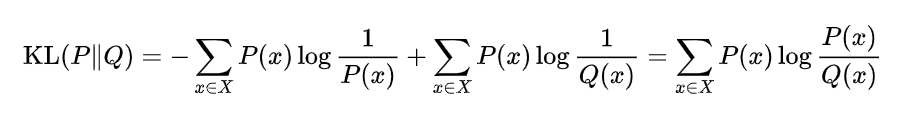4.互信息
两个随机变量X和Y的互信息，定义为X，Y的联合分布和独立分布乘积的相对熵。即：所以根据KL散度也就是相对熵的定义，可以推出互信息的表达式如下：继续推导如下：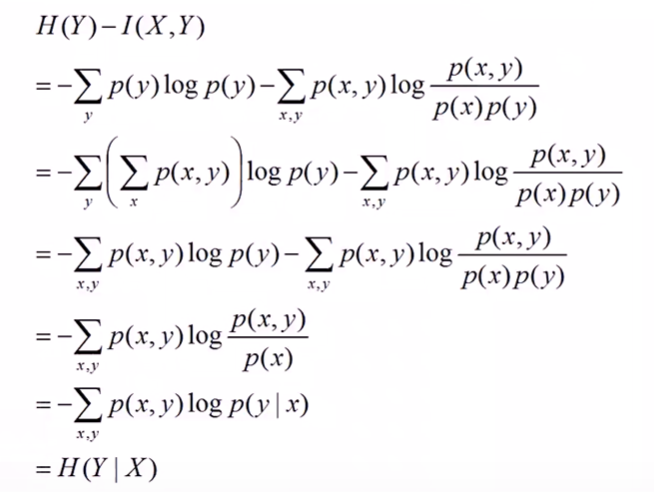所以最后有：5.几个量之间的关系
结合上述条件熵的两个表达式，可以进一步推出：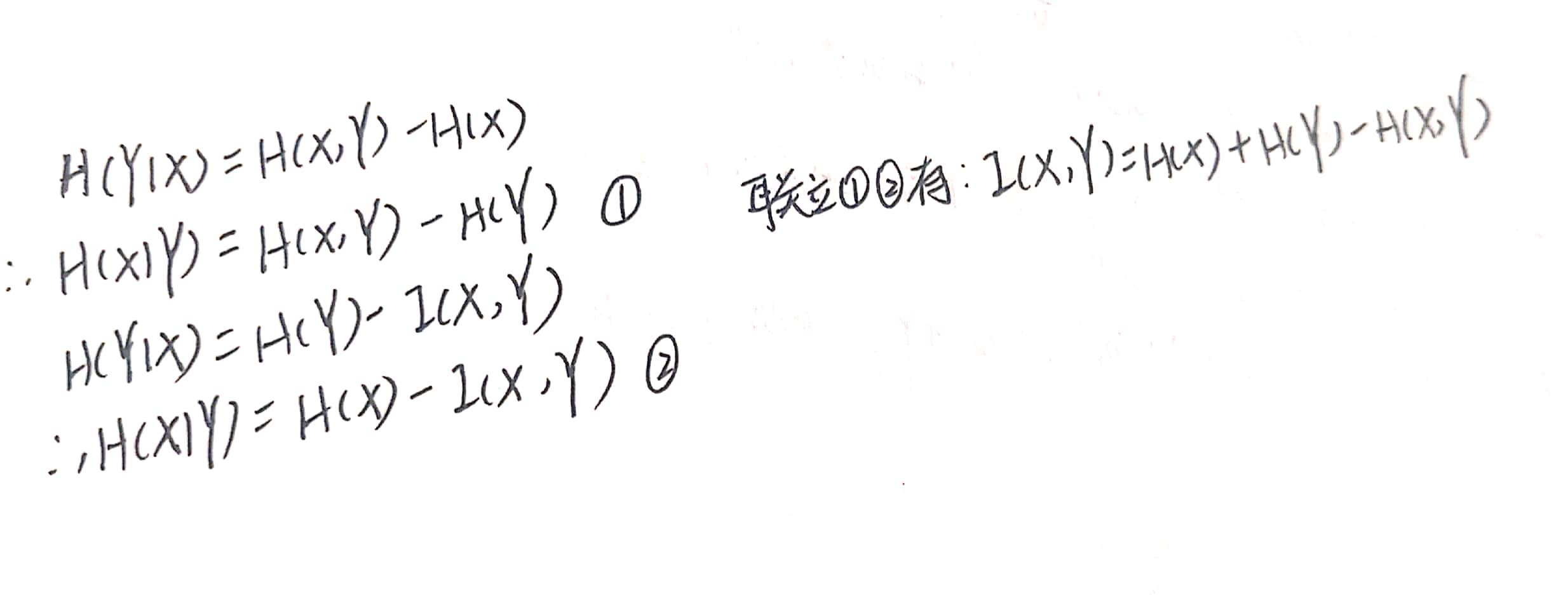当然我们也可以根据熵的定义来直接推出上面这个互信息的公式：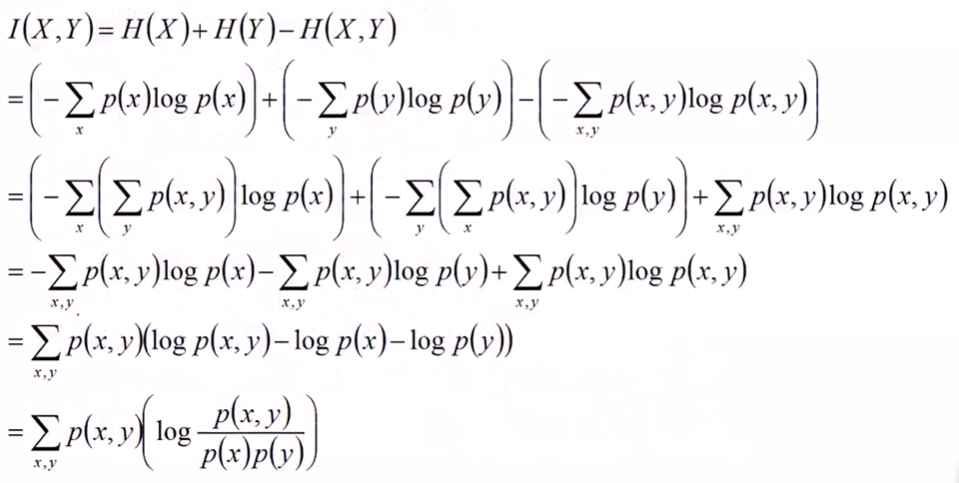同时我们也可以得到两个不等式：上面这个不等式告诉我们，对于一个与X相关的随机变量Y，只要我们得知了一点关于Y的信息，那么X的不确定度就会减小。   最后，借助强大的韦恩图来记住这些关系：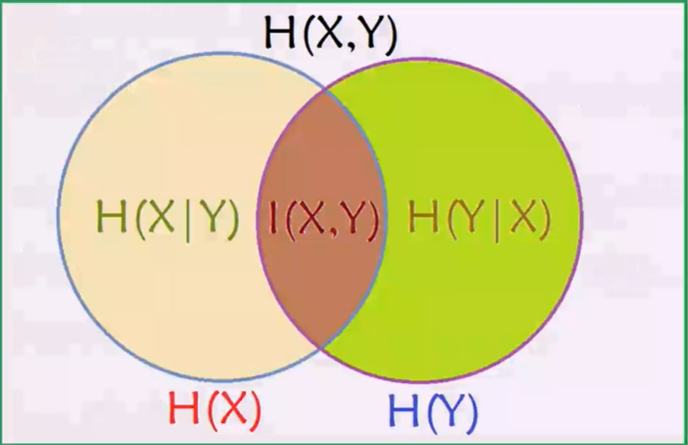二、决策树
1.引入
决策树，顾名思义，就是帮我们做出决策的树。现实生活中我们往往会遇到各种各样的抉择，把我们的决策过程整理一下，就可以发现，该过程实际上就是一个树的模型。比如相亲的时候：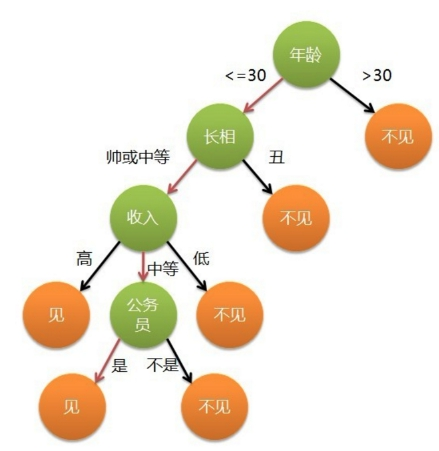我们可以认为年龄，长相，收入是一个人的三个特征，每次我们做出抉择都是基于这三个特征来把一个节点分成好几个新的节点。   一颗完整的决策树包含以下三个部分：
根节点：就是树最顶端的节点，比如上面图中的“年龄”。叶子节点：树最底部的那些节点，也就是决策结果，见还是不见。内部节点，除了叶子结点，都是内部节点。
树中每个内部节点表示在一个属性特征上的测试，每个分支代表一个测试输出，每个叶节点表示一种类别。 给定一个决策树的实例：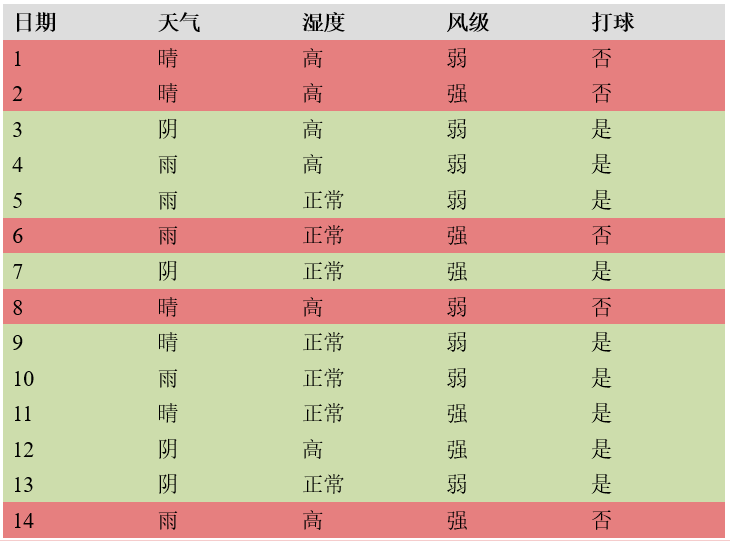构造决策树如下：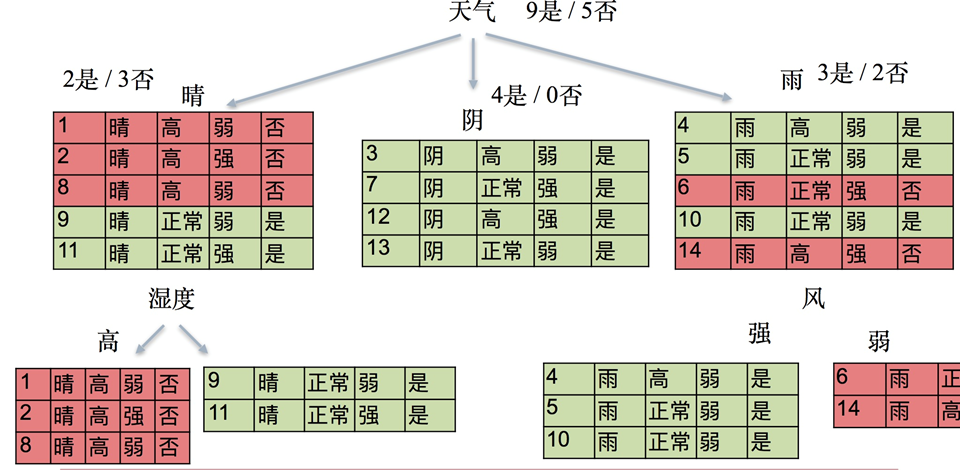1.第一层根节点 被分成14份，9是/5否，总体的信息熵为： H0= -[(5/14)log5/14+(9/14)log9/14]=0.9403.
2.第二层 晴：被分为5份，2是/3否，它的信息熵为： H1=-[(2/5)log2/5+(3/5)log3/5]=0.9710. 阴：被分为4份，4是/0否，它的信息熵为： H2= -[log1]=0. 雨：被分为5份，3是/2否，它的信息熵为： H3=-[(2/5)log2/5+(3/5)log3/5]=0.9710.    我们规定，假设我们选取天气为分类依据，把它作为根节点，那么第二层的加权信息熵，也就是

H

′

=

5

/

14

H

1

+

4

/

14

H

2

+

5

/

14

H

3

H^{'}=5/14H1+4/14H2+5/14H3

要比H0小，也就是随着决策的进行，其不确定度要减小才行，要不然我们还决策干什么。。。决策肯定是一个由不确定到确定状态的转变。 算出

H

′

=

0.6936

<

0.9403

H^{'}=0.6936<0.9403

，符合要求。    事实上，随着分类的进行，越来越多的信息被知道，那么总体的熵肯定实惠下降的。   同样，对于晴这个节点，他的两个叶子结点的熵都是0，到了叶子结点之后，熵就变为0了，就得到了决策结果。    因此，决策树采用的是自顶向下的递归方法，其基本思想是以信息熵为度量构造一棵熵值下降最快的树，到叶子节点处的熵值为0，此时每个叶子节点中的实例都属于同一类。 至于怎么定义下降最快，下面接着讲。
2.决策树的生成算法
首先我们要选择一个根节点，那么选谁当做根节点呢？比如上面的例子，有天气，湿度以及风级三个属性，所以我们要在三个当中选择一个。三个属性f1,f2,f3，以三个属性分别为根节点可以生成三棵树（从第一层到第二才层），而究竟选择谁来当根节点的准则，有以下三种。
1.信息增益与ID3
决策树中信息增益定义如下：   给定一个样本集D，划分前样本集合D的熵是一定的 ，用H0表示； 使用某个特征A划分数据集D，计算划分后的数据子集的熵，用H1表示，则：   信息增益=H0-H1，也可以表示为：比如上面实例中我选择天气作为根节点，将根节点一分为三，设f1表示天气，则有:

g

(

D

,

f

1

)

=

H

0

−

H

′

=

0.9403

−

0.6936

=

0.2467.

g(D,f1)=H0-H^{'}=0.9403-0.6936=0.2467.

意思是，没有选择特征f1前，是否去打球的信息熵为0.9403，在我选择了天气这一特征之后，信息熵下降为0.6936，信息熵下降了0.2467，也就是信息增益为0.2467.
信息增益的局限：信息增益偏向取值较多的特征。   原因：当特征的取值较多时，根据此特征划分更容易得到纯度更高的子集，因此划分之后的熵更低，由于划分前的熵是一定的，因此信息增益更大，因此信息增益比较偏向取值较多的特征。 比如说有一个特征可以把训练集的每一个样本都当成一个分支，也就说有n个样本，该特征就把树分成了n叉树，那么划分后的熵变为0，因此信息增益当然是下降最大的。 也就是如果我们在生成决策树的时候以信息增益作为判断准则，那么分类较多的特征会被优先选择。   利用信息增益作为选择指标来生成决策树的算法称为ID3算法。
2.信息增益率与C4.5
为了解决信息增益的局限，引入了信息增益率的概念。分支过多容易导致过拟合，造成不理想的后果。假设原来的熵为0.9，选择f1特征划分后整体熵变成了0.1，也就是信息增益为0.8，而选择f2划分后，熵变为0.3，也就是信息增益为0.6。我们不想选择f1，因为它让决策树分支太多了，那么就可以定义决策指标=信息增益/特征本身的熵。f1划分后分支更多，也就是特征f1本身的熵比f2更大，大的数除以一个大的数，刚好可以中和一下。   即：还是以这张图为例子算一下：现在我们要选择谁做根节点。未划分前：（前面都算过一遍了） H0= 0.9403. 选择天气作为根节点，则有： 晴：被分为5份，2是/3否，它的信息熵为： H1=0.9710. 阴：被分为4份，4是/0否，它的信息熵为： H2= -[log1]=0. 雨：被分为5份，3是/2否，它的信息熵为： H3=0.9710. 划分后总体的信息熵为：

H

′

=

5

/

14

H

1

+

4

/

14

H

2

+

5

/

14

H

3

=

0.6936

H^{'}=5/14H1+4/14H2+5/14H3=0.6936

。一定要注意，这个算的是总体的信息熵， 那么信息增益为：0.2467. 这个时候我们考虑天气本身的熵，这里算的是天气本身的熵，而不是样本X,也就是是否外出打球的熵，这里一定要将二者区分开。天气本身有三种可能，每种概率都已知，则天气的熵为：

H

0

=

−

[

(

5

/

14

)

l

o

g

5

/

14

+

(

4

/

14

)

l

o

g

4

/

14

+

(

5

/

14

)

l

o

g

5

/

14

]

=

0.9403

=

1.5774

H0= -[(5/14)log5/14+(4/14)log4/14+(5/14)log5/14]=0.9403=1.5774

. 那么选择天气作为分类依据时，评价指标=0.2467/1.5774=0.1566.
这里还是想要强调一下，很多人在算信息增益率的时候总是出错，是因为搞混了总体的熵与特征的熵！！！ 我在算信息增益的时候，两个相减的熵都是算的总体的熵，也就是依据是否外出来划分的，而我计算特征本身的熵的时候，是依据这个特征本身来算的，比我算天气的熵，那么就看天气分为了哪几类，比如晴天，阴天，雨天之类的，每一类又有自己的概率，然后再根据信息熵的定义来计算，二者相除即是信息增益率。   利用信息增益率作为选择指标来生成决策树的算法称为C4.5算法。
3.Gini系数与CART
定义：基尼指数（基尼不纯度）：表示在样本集合中一个随机选中的样本被分错的概率。   定义式：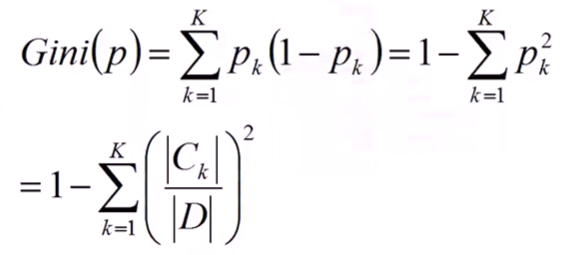一些参数的说明：
pk表示选中的样本属于k类别的概率，则这个样本被分错的概率是(1-pk)。样本集合中有K个类别，一个随机选中的样本可以属于这k个类别中的任意一个。易知，当样本属于每一个类别的概率都相等即均为1/K时，基尼系数最大，也就是说此时不确定度最小。
关于基尼系数的理解，网上有一种说法比较通俗易懂。现解释如下：   我们知道信息熵的定义式为：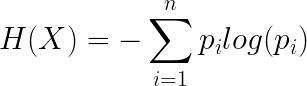那么基尼系数实际上就是用

1

−

p

i

1-p_{i}

来代替了

−

l

o

g

(

p

i

)

-log(p_{i})

，画出二者图像：因为概率是属于0到1之间，所以我们只看01区间上的图像：基尼系数对于信息熵而言，就是在01区间内近似的用切线来代替了对数函数。因此，既然信息熵可以表述不确定度，那么基尼系数自然也可以，只不过存在一些误差。
CART决策树又称分类回归树，当数据集的因变量为连续性数值时，该树算法就是一个回归树，可以用叶节点观察的均值作为预测值；当数据集的因变量为离散型数值时，该树算法就是一个分类树，可以很好的解决分类问题。   当CART是分类树时，采用GINI值作为结点分裂的依据；当CART是回归树时，采用MSE(均方误差)作为结点分裂的依据。我们这里只讨论分类。
3.决策树的评价
假定样本总类别为K,而对于决策树的某叶节点，假定该叶子节点包含的样本数目为n，其中第k类的样本数目为

n

k

n_{k}

，k=1,2,…,K。 若某类样本的

n

j

n_{j}

=n，其他类别个数全为0，也就是说该叶结点中所有样本完全属于同一个类，则称该结点为纯结点，其熵为0.   若某一叶子结点中包含了所有类别的样本且各类数目相同，则称该结点为均结点。 其熵为

l

o

g

K

logK

。   那么我们对所有叶子结点的熵求和，该值越小说明越精确。 而又由于各个叶子结点包含的样本数目不同，所以我们采用加权熵和。   评价函数定义如下：其中

N

t

N_{t}

表示叶子结点的样本数目，评价函数越小说明决策树越好。
4.决策树的过拟合
当决策树深度过大时，在训练集上表现特别好，往往就会出现过拟合现象，我们需要一些解决办法：
1.剪枝
剪枝总体思路：    由完全树T0开始，剪枝部分结点得到树T1，然后再剪枝部分结点得到树T2，…，直到仅剩树根的Tk。在验证数据集上对这K个树分别评价，选择损失函数最小的树

T

α

T_{α}

。    三种决策树的生成算法过程相同，只是对于当前树的评价标准不同。
三、随机森林
随机森林也是为了解决决策树的过拟合问题。
1.Bootstrap
假设有一个大小为N的样本，我们希望从中得到m个大小为N的样本用来训练。bootstrap的思想是：首先，在N个样本里随机抽出一个样本x1，然后记下来，放回去，再抽出一个x2，… ，这样重复N次，即可得到N个新样本，这个新样本里可能有重复的。重复m次，就得到了m个这样的样本。实际上就是一个有放回的随机抽样问题。每一个样本在每一次抽的时候有同样的概率（1/N）被抽中。
2.bagging策略
bagging的名称来源于： Bootstrap Aggregating，意为自助抽样集成。既然出现了Bootstrap那么肯定就会使用到Bootstrap方法，其基本策略是：
利用Bootstrap得到m个样本大小为N的样本集。在所有属性上，对每一个样本集建立分类器。将数据放在这m个分类器上，最后根据m个分类器的投票结果，决定数据最终属于哪一类。如果是回归问题，就采用均值。
什么时候用bagging？当模型过于复杂容易产生过拟合时，才使用bagging，决策树就容易产生过拟合。
3.out of bag estimate（包外估计）
在使用bootstrap来生成样本集时，由于我们是有放回抽样，那么可能有些样本会被抽到多次，而有的样本一次也抽不到。我们来做个计算：假设有N个样本，每个样本被抽中的概率都是1/N，没被选中的概率就是1-1/N，重复N次都没被选中的概率就是

(

1

−

1

/

N

)

N

(1-1/N)^N

，当N趋于无穷时，这个概率就是1/e，大概为36.8%。也就是说样本足够多的时候，一个样本没被选上的概率有36.8%，那么这些没被选中的数据可以留作验证集。每一次利用Bootstrap生成样本集时，其验证集都是不同的。   以这些没被选中的样本作为验证集的方法称为包外估计。
4.样本随机与特征随机
在我们使用Bootstrap生成m个样本集时，每一个样本集的样本数目不一定要等于原始样本集的样本数目，比如我们可以生成一个含有0.75N个样本的样本集，此处0.75就称为采样率。   同样，我们在利用0.75N个样本生成决策树时，假设我们采用ID3算法，生成结点时以信息增益作为判断依据。我们的具体做法是把每一个特征都拿来试一试，最终信息增益最大的特征就是我们要选的特征。但是，我们在选择特征的过程中，也可以只选择一部分特征，比如20个里面我只选择16个特征。   那可能有的人就要问了，假设你没选的4个特征里面刚好有一个是最好的呢？这种情况是完全可能出现的，但是我们在下一次的分叉过程中，该特征是有可能被重新捡回来的，另外别的决策树当中也可能会出现那些在另一颗决策树中没有用到的特征。   随机森林的定义就出来了，利用bagging策略生成一群决策树的过程中，如果我们又满足了样本随机和特征随机，那么构建好的这一批决策树，我们就称为随机森林(Random Forest)。   实际上，我们也可以使用SVM，逻辑回归等作为分类器，这些分类器组成的总分类器，我们习惯上依旧称为随机森林。`
展开全文基尼系数 机器学习 信息熵
• https://www.shiyanlou.com/courses/863 参考教程决策树进行鸢尾花分类使用决策树一些关键步骤： 特征选取：信息增益越大，该特征越重要 节点划分算法： ID3，利用信息增益进行节点划分，选取信息增益最大的特征...
• 决策树：如何用决策树选择相亲对象 读书笔记： 管理就是决策决策树就是种把决策节点...四个步骤1、邀请专家；2、独立预测；3、统计回归；4、分析结果； 注意两点：1、必须避免专家们面对面集体讨论，而要...德尔菲法 麦穗理论
• 决策树是种基本的分类和回归方法。决策树呈树形结构，在分类问题中，表示基于特征对实例进行分类的过程。...决策树学习通常包括三个步骤：特征选择、决策树的生成和决策树的剪枝。（ID3、C4.5、CART）统计学习方法
• 数据挖掘有很多不同的实施方法，如果...从数据本身来考虑，数据挖掘通常需要有信息收集、数据集成、数据规约、数据清理、数据变换、数据挖掘实施过程、模式评估和知识表示8个步骤步骤1）信息收集：根据确定的数大数据
• 选择适合某个产品使用的微处理器是一项艰巨的任务。不仅要考虑许多技术因素，而且要考虑可能影响到项目成败的成本和交货时间等商业问题。 　在项目刚启动时，人们经常压抑不住马上动手的欲望，在系统细节...第一种是通
• 本篇是我学习决策树的一个入门，主要介绍最经典的决策树算法 ID3 决策树是由昆兰提出的种作为分类的算法；它的特点主要是形象，简单易懂，不需要较多的专业领域知识；缺点则是根据不同算法各有不同；本篇先介绍最...机器学习 算法
• ## 决策树

千次阅读 2019-03-05 10:14:48
通常决策树的学习分为3...决策树的本质是树，对应上面具体的问题，构建树的时候，选择年龄、有工作、有自己的房子、信贷情况、他们中谁作为二叉树的第一个节点会让分类的结果准确一点。就产生了决策树的特征选择...
• (decision tree) 是种分类与回归方法，主要用于分类，决策树模型呈现树形结构，是基于输入特征对实例进行分类的模型。我认为决策树其实是定义在特征空间与类空间上的条件概率分布！ 使用决策树算法的主要步骤又...机器学习
• 第一步：适合应用的文档一般是确定的大量文本数据。文档聚类方法经常用语解决“大量”这问题。这些方法是非监督的学习方法，最受欢迎的文档聚类方法是K-means聚类和凝聚层次聚类。 第二步：文本是被清洗了的——它...文本挖掘
• ## 【自考}运筹学 第三章决策

千次阅读 热门讨论 2015-02-12 10:53:49
本章讲解的内容围绕两主题：...决策步骤为：1、确定目标；2、拟定多可行方案；3、预测可能发生的自然状态，计算不同方案在不同的自然状态下的收益值，编制决策收益表；4、以决策收益表为根据，运用不同的决策标砖
• 决策树（decision tree） 说明：这篇博客是看周志华老师的《机器学习》（西瓜书）的笔记总结，博客中有大段文字摘自周老师的《机器学习》书，仅供学习交流使用。转载博客务必注明出处和作者，谢谢。 决策树算法起源...信息增益 机器学习
• 四章：决策树4.1 基本流程4.1.1 决策树的结构4.1.2 决策树学习基本算法4.2 划分选择4.2.1 决策树学习的关键4.2.2 信息增益(information gain)信息熵属性a的信息增益$Gain(D,a)$4.2.3 增益率(gain ratio)4.2.4 基尼...西瓜书 最优划分属性 信息熵
• ## 决策树介绍

千次阅读 2018-06-30 23:13:50
决策树的学习过程主要包括3个步骤：特征选择、决策树的生成和决策树的剪枝。常用的算法有ID3算法、C4.5算法以及CART算法。后面会一一介绍这些算法。1.决策树模型 决策树定义：分类决策树模型是种描述对实例进行...机器学习
• 决策决策树是种基本的分类与回归算法。 在分类问题中，表示基于...决策树学习通常包括三个步骤：特征选择、决策树的生成和决策树的修剪。 决策树的算法通常是递归地选择最优特征，并根据该特征对训练数据...
• 3章 决策树 http://cdn.mathjax.org/mathjax/latest/MathJax.js?config=default">> 决策树 概述 决策树（Decision Tree）算法主要用来处理分类问题，是最经常使用的数据挖掘算法之决策树 场景 一个...判定鱼类和非鱼类 机器学习实战
• ## 决策表

千次阅读 2017-10-16 10:56:32
例如下图，按右半部第一列的规则看，当是普通会员没有特别折扣时触发无折扣的动作。 开发决策表的步骤： 列出特定过程（或构件）相关的所有动作。列出执行该过程时所有的条件（或所做的决策）。将特定的条件组合与...
• 前言博主第一次接触机器学习，内容可能有许多原文复现，但是我尽量用自己的话来讲读书笔记第一目的是为了总结，第二是顺便在博客上记录我的学习历程，同时也希望读者能有一点点收获吧~如果不对的地方，还请多多指教...机器学习
• ## 第5节--决策树算法

千次阅读 2016-10-21 11:25:04
1、什么是决策树（decision tree）决策树是一个类似于流程图的树结构：其中，每内部结点表示在一个属性上的测试，每分支代表一个属性输出，而每树叶结点代表类或类分布。树的最顶层是根结点。2、决策树算法是...判定树 ID3 机器学习算法...